In: Math

# (1 point) Let F=−3yi+4xjF=−3yi+4xj. Use the tangential vector form of Green's Theorem to compute the circulation...

(1 point) Let F=−3yi+4xjF=−3yi+4xj. Use the tangential vector form of Green's Theorem to compute the circulation integral ∫CF⋅dr∫CF⋅dr where C is the positively oriented circle x2+y2=25x2+y2=25.

## Solutions

##### Expert Solution

Solution: Given a vector function F: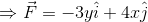Now, by using the tangential form of the green's theorem, we've to calculate the circulation given by: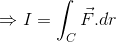Here C is positively oriented curve given by: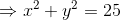To do this, we should know the concept of Green's theorem (tangential-form).

Green's theorem: Let C be a piecewise smooth, simple closed curve in the plane and D be the region inside the enclosed by the curve C. If F is a vector field such that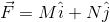Where M and N are functions of x and y and having continuous partial derivatives in the region D, then according to the green's theorem: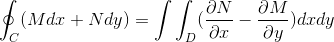...(1)

Here, the integral is transversed in the counter-clockwise direction

Now, in our case, the vector function and curve C is given by:Since D is the region enclosed by the curve C, therefore, it will be: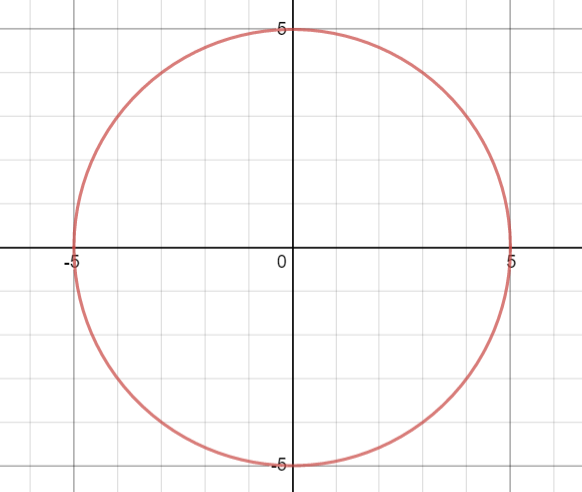We've to calculate:Since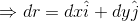Therefore,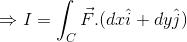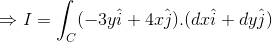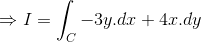Now, applying the tangential form of the green's theorem, we'll get: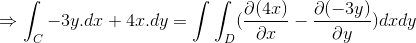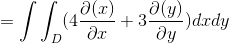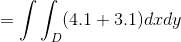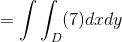In the graph of the region D above, we can see that the value of x and y varies between: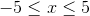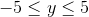Therefore, our integration becomes: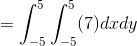Using standard integration: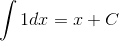We'll get: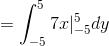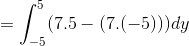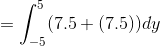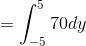Using standard integration: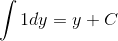We'll get: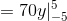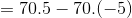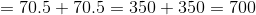​​​​​​​I hope it helps you!

## Related Solutions

##### Use Stokes' theorem to compute the circulation F · dr where F = 8xyz, 2y2z, 5yz...
Use Stokes' theorem to compute the circulation F · dr where F = 8xyz, 2y2z, 5yz and C is the boundary of the portion of the plane 2x + 3y + z = 6 in the first octant. Here C is positively oriented with respect to the plane whose orientation is upward.
##### Problem 1: Use Green's Theorem to evaluate the vector line integral ∫C [?3??−?3] ?? where ?...
Problem 1: Use Green's Theorem to evaluate the vector line integral ∫C [?3??−?3] ?? where ? is the circle ?2+?2=1 with counterclockwise orientation. Problem 2: Which of the following equations represents a plane which is parallel to the plane 36?−18?+12?=30 and which passes through the point (3,6,1) ? a). 6?−3?+2?=3 b). 6?+3?−2?=34 c). 36?+18?−12?=204 d). 6?−3?+2?=2 e). 36?+18?+12?=228
##### Use the extended divergence theorem to compute the total flux of the vector field F(x, y,...
Use the extended divergence theorem to compute the total flux of the vector field F(x, y, z) = −3x2 + 3xz − y, 2y3 − 6y, 9x2 + 4z2 − 3x outward from the region F that lies inside the sphere x2 + y2 + z2 = 25 and outside the solid cylinder x2 + y2 = 4 with top at z = 1 and bottom at z = −1.
##### Theorem: Let K/F be a field extension and let a ∈ K be algebraic over F....
Theorem: Let K/F be a field extension and let a ∈ K be algebraic over F. If deg(mF,a(x)) = n, then 1. F[a] = F(a). 2. [F(a) : F] = n, and 3. {1, a, a2 , ..., an−1} is a basis for F(a).
##### Prove the theorem in the lecture:Euclidean Domains and UFD's Let F be a field, and let...
Prove the theorem in the lecture:Euclidean Domains and UFD's Let F be a field, and let p(x) in F[x]. Prove that (p(x)) is a maximal ideal in F[x] if and only if p(x) is irreducible over F.
##### Verify that the Divergence Theorem is true for the vector field F on the region E....
Verify that the Divergence Theorem is true for the vector field F on the region E. Give the flux. F(x, y, z) = xyi + yzj + zxk, E is the solid cylinder x2 + y2 ≤ 144, 0 ≤ z ≤ 4.
##### Calculate integlar c F(r)dr when F =[xlny, ye^x] using Green's theorem. R is a rectangle whose...
Calculate integlar c F(r)dr when F =[xlny, ye^x] using Green's theorem. R is a rectangle whose vertices are (0,1), (3,1), (3,2), (0,2).
##### Let F and G~be two vector fields in R2 . Prove that if F~ and G~...
Let F and G~be two vector fields in R2 . Prove that if F~ and G~ are both conservative, then F~ +G~ is also conservative. Note: Give a mathematical proof, not just an example.
##### (3) Let V be a vector space over a field F. Suppose that a ? F,...
(3) Let V be a vector space over a field F. Suppose that a ? F, v ? V and av = 0. Prove that a = 0 or v = 0. (4) Prove that for any field F, F is a vector space over F. (5) Prove that the set V = {a0 + a1x + a2x 2 + a3x 3 | a0, a1, a2, a3 ? R} of polynomials of degree ? 3 is a vector space over...
##### Verify the divergence theorem for the vector ﬁeld F = 2xzi + yzj +z2k and V...
Verify the divergence theorem for the vector ﬁeld F = 2xzi + yzj +z2k and V is the volume enclosed by the upper hemisphere x2 + y2 + z2 = a2, z ≥ 0